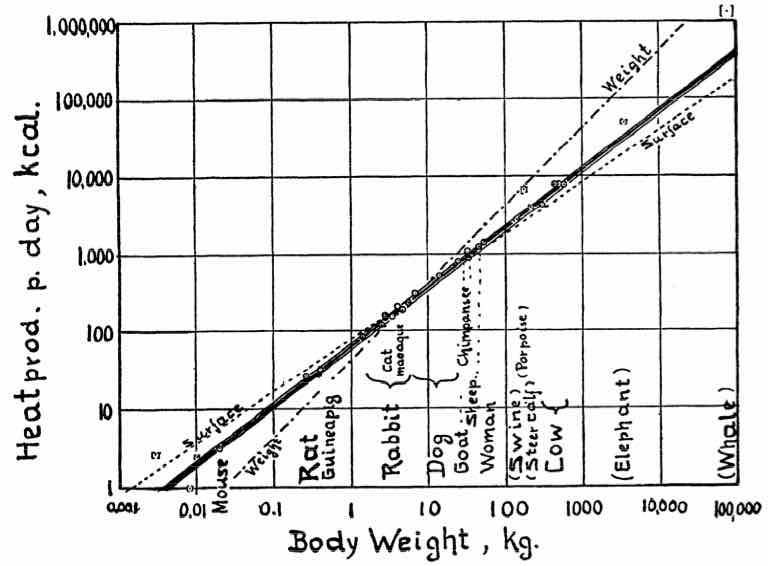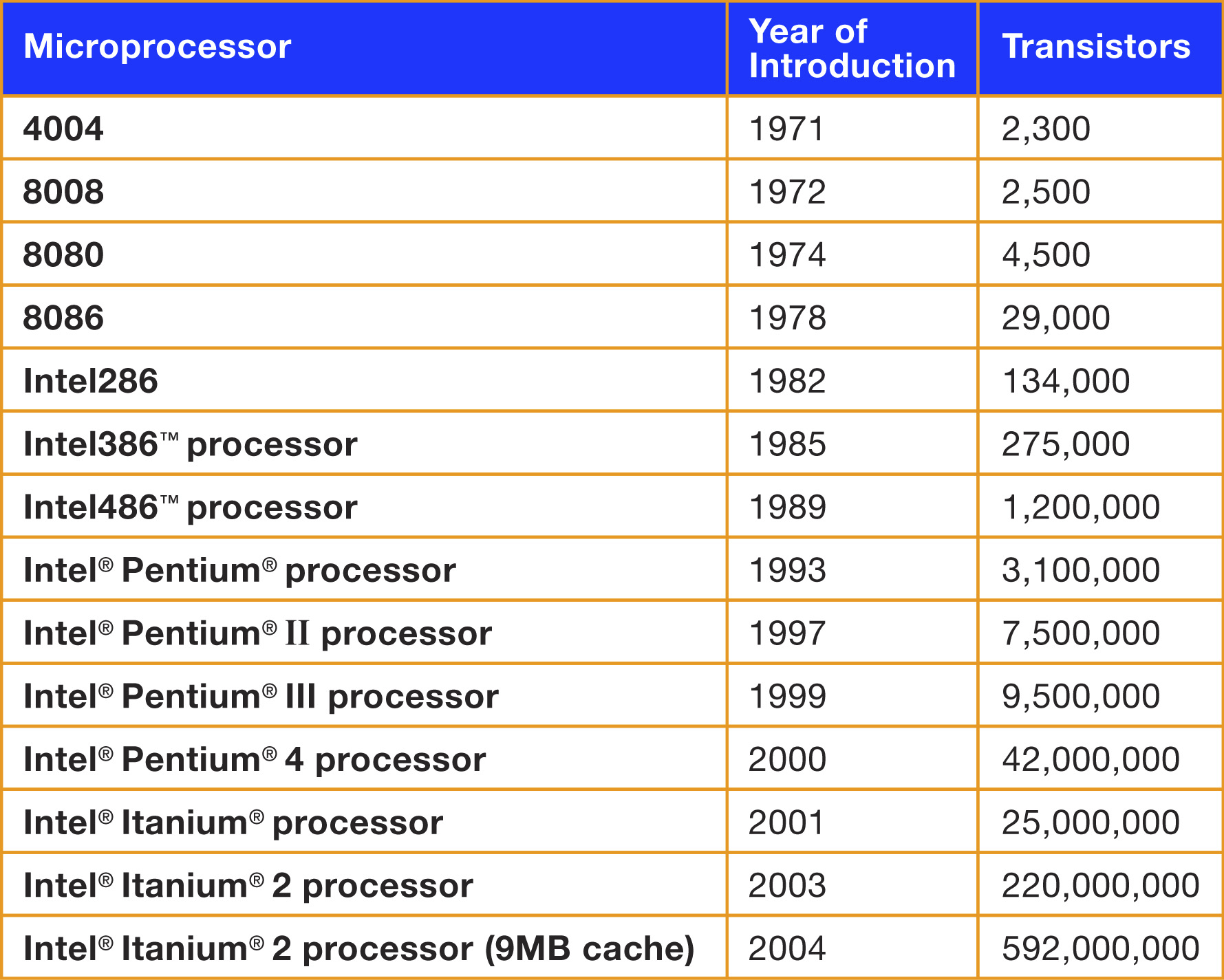December 10, 2019

## What is a logarithm?

We need very little math for our course: arithmetic, algebra, and logarithms

Just remember that if $$x=p^m$$ then $\log_p(x) = m$

If we use another base, for example $$q$$, then $\log_q(x) = m\cdot\log_q(p)$

So if we use different bases, there is only a scale factor

The easiest one is natural logarithm

• They only work with positive numbers. Not with 0
• If $$x=p\cdot q$$ then $\log(x)=\log(p)+\log(q)$
• If $$x=a^m$$ then $\log(x)=m\log(a)$
• If $$x=\exp(m)$$ then $\log(x)=m$

## Linear models can be used in three cases

Basic linear model $y=A+B\cdot x$ Exponential $y=I\cdot R^x\qquad\log(y)=log(I)+log(R)\cdot x$ Power of $$x$$ $y=C\cdot x^E\qquad\log(y)=log(C)+E\cdot\log(x)$

## Which one to use?

The easiest way to decide is to

• draw several plots, placing log() in different places,
• see which one seems more like a straight line

For example, let’s analyze data from Kleiber’s Law

The following data shows a summary. The complete table has 26 animals

## Body size v/s metabolic rate

animal kg kcal
Mouse 0.021 3.6
Rat 0.282 28.1
Guinea pig 0.410 35.1
Rabbit 2.980 167.0
Cat 3.000 152.0
Macaque 4.200 207.0
Dog 6.600 288.0
animal kg kcal
Goat 36.0 800
Chimpanzee 38.0 1090
Sheep ♂ 46.4 1254
Sheep ♀ 46.8 1330
Woman 57.2 1368
Cow 300.0 4221
Young cow 482.0 7754

## First plot: Linear

plot(kcal ~ kg, data=kleiber)## Second plot: semi-log

plot(log(kcal) ~ kg, data=kleiber)## Third plot: log-log

plot(log(kcal) ~ log(kg), data=kleiber)## Which one seems more “straight”?

The plot that seems more straight line is the log-log plot

Therefore we need a log-log model.

model <- lm(log(kcal) ~ log(kg), data=kleiber)
coef(model)
(Intercept)     log(kg)
4.206       0.756 

## What is the interpretation of these coefficients?

If $\log(kcal)=4.21 + 0.756\cdot \log(kg)$ then $kcal=\exp(4.21) \cdot kg^{0.756} =67.1 \cdot kg^{0.756}$

Therefore:

• For a 1kg animal, the average energy consumption is $$\exp(4.21) = 67.1$$ kcal
• The energy consumption increases at a rate of $$0.756$$ kcal/kg.

## This is Kleiber’s Law

“An animal’s metabolic rate scales to the ¾ power of the animal’s mass”.

## Two ways to do logarithmic scales

Depending on the goal, we use different versions of semi-log and log-log plots

For understanding the data, we do

plot(log(kcal) ~ kg, data=kleiber)

For publishing in a paper, we do

plot(kcal ~ kg, data=kleiber, log="y")

## Example

plot(log(kcal) ~ kg,
data=kleiber)plot(kcal ~ kg,
data=kleiber, log="y")## Example

plot(log(kcal) ~ log(kg),
data=kleiber)plot(kcal ~ kg,
data=kleiber, log="xy")## What can we do with the model?

Models are the essence of scientific research

They provide us with two important things

• An explanation for the observed patterns of nature
• A method to predict what will happen in the future

## Predicting with the model

predict(model, newdata)

where newdata is a data frame with column names corresponding to the independent variables

If we omit newdata, the prediction uses the original data as newdata

predict(model) == predict(model, newdata=data)

## Results

### What is wrong here?

animal kg kcal predicted
Mouse 0.021 3.6 1.28
Rat 0.282 28.1 3.25
Guinea pig 0.410 35.1 3.53
Rabbit 2.980 167.0 5.03
Cat 3.000 152.0 5.04
Macaque 4.200 207.0 5.29
Dog 6.600 288.0 5.63
animal kg kcal predicted
Goat 36.0 800 6.92
Chimpanzee 38.0 1090 6.96
Sheep ♂ 46.4 1254 7.11
Sheep ♀ 46.8 1330 7.11
Woman 57.2 1368 7.26
Cow 300.0 4221 8.52
Young cow 482.0 7754 8.88

## Undoing the logarithm

We want to predict the metabolic rate, depending on the weight

The independent variable is $$kg$$, the dependent variable is $$kcal$$

But our model uses only $$\log(kg)$$ and $$\log(kcal)$$

So we have to undo the logarithm, using $$\exp()$$

## Correct formula for prediction

predicted_kcal <- exp(predict(model))
animal kg kcal predicted
Mouse 0.021 3.6 3.62
Rat 0.282 28.1 25.76
Guinea pig 0.410 35.1 34.19
Rabbit 2.980 167.0 153.11
Cat 3.000 152.0 153.89
Macaque 4.200 207.0 198.46
Dog 6.600 288.0 279.29
animal kg kcal predicted
Goat 36.0 800 1007
Chimpanzee 38.0 1090 1049
Sheep ♂ 46.4 1254 1220
Sheep ♀ 46.8 1330 1228
Woman 57.2 1368 1429
Cow 300.0 4221 5001
Young cow 482.0 7754 7157

## Visually

plot(log(kcal) ~ log(kg), data=kleiber)
lines(predict(model) ~ log(kg), data=kleiber)## Visually

plot(kcal ~ kg, data=kleiber, log="xy")
lines(exp(predict(model)) ~ kg, data=kleiber)## In the paper## Moore’s Law## Real data: Number of transistors in chips v/s year

plot(count~Date, data=trans)## Semilog scale: Number of transistors v/s year

plot(log(count) ~ Date, data=trans)## Semi-log means exponential growth

we have straight line on the semi-log

That is, log(y) versus x $\log(y)=log(I)+log(R)\cdot x$ In this case the original relation is $y=I\cdot R^x$

## Model

model <- lm(log(count) ~ Date, data=trans)
exp(coef(model))
(Intercept)        Date
7.83e-295    1.41e+00 

## Semi-log scale and model

plot(count ~ Date, data=trans, log="y")
lines(exp(predict(model)) ~ Date, data=trans)## Meaning

Every year processors grow by a factor of

exp(coef(model))
Date
1.41